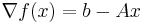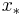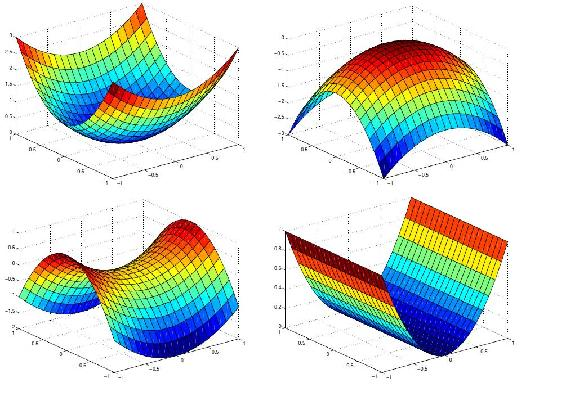# 8.3: Non-linear Optimization

•• Contributed by Peter Woolf et al.
• Assistant Professor (Chemical Engineering) at University of Michigan

Written by: Matthew Goh, Andrew King, and Edwin Yik

## 3.1 Introduction

Various conditions and situations are not adequately described using linear systems. In this case, nonlinear optimization may be applied. Unlike linear optimization, the optimal operating condition does not exist at the boundaries.

$f(x)=c-x^{T} b+\frac{1}{2} x^{T} A x$

To optimize, it is necessary to find when the gradient of f is equal to zero.It may be possible to solve the optimalby a linear equation, approximated by a Taylor series.

$f\left(x_{*}\right)=f(x)+\left(x_{*}-x\right)^{\prime} \nabla f(x)+\frac{1}{2}\left(x_{*}-x\right)^{\prime} \nabla \nabla f(x)\left(x_{*}-x\right)+\ldots$

## 3.3 Iterative Methods

When direct methods cannot solve the equation (i.e. A is not symmetric positive definite), iterative methods are possible .

By starting with an initial guess of, an algorithm may lead to athat better satisfies the equation. Through iteration, theoretically,.

## 3.4 Applications

• Finance: Portfolio optimization
• Engineering: Rigid body dynamics
• Biochemistry: Kinetic modeling 

## 3.5 Example: Typical Nonlinear 3d Curves(Image from )

As observed, the optimal condition does not necessarily exist at the boundary of the curve.

$f(x)=\vec{c}^{T} \vec{x}+\frac{1}{2} \vec{x}^{T} Q \vec{x}$

where

$\vec{c}^{T}=\left(c_{1}, c_{2}, \ldots, c_{n}\right)$

$\vec{x}^{T}=\left(x_{1}, x_{2}, \ldots, x_{n}\right)$

For a quadratic system, $$n=2$$, thus, $$Q$$ (the quadratic term constant) is defined as a symmetric matrix as follows.

$Q=\left[\begin{array}{ll} Q_{1} & Q_{3} \\ Q_{3} & Q_{2} \end{array}\right]$

Thus, multiplying out the $$f$$,

$f(x)=\left(c_{1} x_{1}+c_{2} x_{2}\right)+\frac{1}{2}\left(Q_{1} x_{1}^{2}+2 Q_{3} x_{1} x_{2}+Q_{2} x_{2}^{2}\right)$

## References

1. Lippert, Ross A. "Introduction to non-linear optimization." D.E. Shaw Research, February 25, 2008. http://www.mit.edu/~9.520/spring08/Classes/optlecture.pdf
2. Mendes, Pedro and Kell, Douglas B. "Non-linear optimization of biochemical pathways: application to metabolic engineering and parameter estimation." Journal of Bioinformatics, Volume 14, 869-883. 1998.
3. "Introduction to Non-linear optimization." Georgia Institute of Technology Systems Realization Laboratory. www.srl.gatech.edu/education/ME6103/NLP-intro.ppt